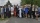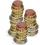# Multiplication principle + prime numbers - math problems

#### Number of problems found: 7

• Wedding guestsFifteen wedding guests could not agree on who would stand in the wedding photo. The groom suggested that all possible sets of wedding guests be made in the photographs.
• Twenty-fiveHow many are three-digit natural numbers divisible by 25?
• Inverted nineIn the hotel Inverted Nine, each hotel room number is divisible by 6. How many rooms can we count with the three-digit number registered by digits 1,8,7,4,9?
• CombinationsHow many different combinations of two-digit number divisible by 4 arises from the digits 3, 5 and 7?
• StacksAnnie has a total of \$ 702. The money must be divided into stacks so that each buyer has the same amount of dollars. How many options she has?
• No. of divisorsHow many different divisors have number 13 4 * 2 4?
• RectanglesHow many rectangles with area 8713 cm2 whose sides are natural numbers are?

We apologize, but in this category are not a lot of examples.
Do you have an exciting math question or word problem that you can't solve? Ask a question or post a math problem, and we can try to solve it.

We will send a solution to your e-mail address. Solved examples are also published here. Please enter the e-mail correctly and check whether you don't have a full mailbox.

Multiplication principle - math problems. Prime numbers - math problems.